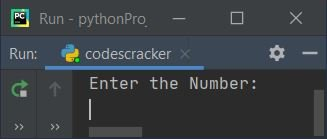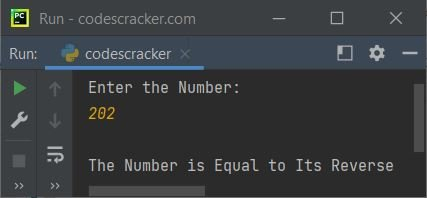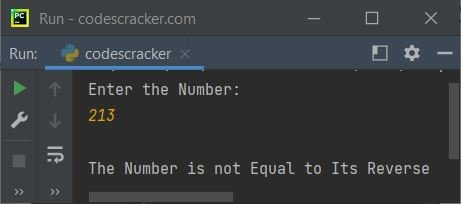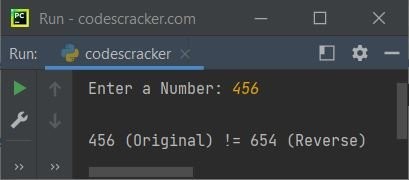# Python Program to Check Reverse Equals Original

In this article, we've created a program in Python that checks whether a number entered by user at run-time is equal to its reverse or not.

Note - To check for palindrome number, refer to Python Program to Check Palindrome Number

## Check Reverse Equals Original in Python

To check whether the original number (entered by user) is equal to its reverse or not in Python, you have to ask from user to enter the number, then check and print the message as shown in the program and its output given below:

```print("Enter the Number: ")
num = int(input())

rev = 0
orig = num
while num>0:
rem = num%10
rev = rem + (rev*10)
num = int(num/10)

if orig==rev:
print("\nThe Number is Equal to Its Reverse")
else:
print("\nThe Number is not Equal to Its Reverse")```

Here is its initial output:Now supply the input say 202 and press `ENTER` key to check whether the given number is equal to its reverse or not. Here is its sample output:Here is another sample run with user input 213:#### Modified Version of Previous Program

This program uses two extra things, that are end and str(). The end is used to skip printing of an automatic newline and str() is used to convert an integer value to a string type value.

```print(end="Enter a Number: ")
n = int(input())

rev = 0
o = n
while n>0:
rem = n % 10
rev = rem + (rev*10)
n = int(n / 10)

if o==rev:
print("\n" +str(o)+ " (Original) = " +str(rev)+ " (Reverse)")
else:
print("\n" +str(o)+ " (Original) != " +str(rev)+ " (Reverse)")```

Here is its sample run with user input 456:#### Same Program in Other Languages

Python Online Test

« Previous Program Next Program »